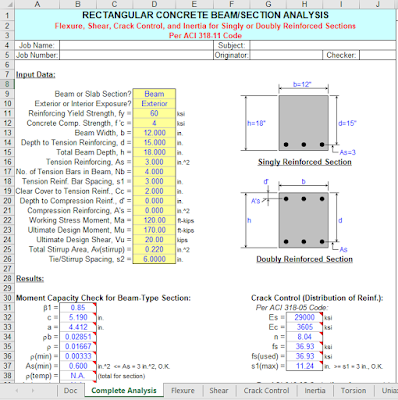# Rectangular Concrete Beam Analysis And Design Spreadsheet

## Rectangular Concrete Beam Analysis And Design

"RECTBEAM" is a spreadsheet program written in MS-Excel for the purpose of analysis/design of rectangular beam or column sections.  Specifically, the ultimate moment capacity, bar spacing for crack control, moments of inertia for deflection, beam shear and torsion requirements, and member capacity for flexure (uniaxial and biaxial) with axial load are calculated.  There is also a worksheet which
contains reinforcing bar data tables. This version is based on the ACI 318-11 Code.
This program utilizes the following references: a. "Design of Reinforced Concrete" (Ninth Edition) (for ACI 318-11), by Jack C. McCormac & Russell H. Brown b. "Notes of the ACI 318-11 Building Code Requirements for Structural Concrete" (Twelfth Edition), by PCA 3.  The "Complete Analysis" worksheet combines the analyses performed by four (4) of the individual worksheets all into one.  This includes member flexural moment capacity, as well as shear, crack control, and inertia calculations.  Thus, any items below pertaining to any of the similar individual worksheets included in this one are also applicable here. 4.  In the "Flexure", "Uniaxial", and "Biaxial" worksheets, when the calculated distance to the neutral axis, 'c', is less than the distance to the reinforcement nearest the compression face, the program will ignore that reinforcing and calculate the ultimate moment capacity based on an assumed singly-reinforced section. 5.  In the "Uniaxial" and "Biaxial" worksheets, the CRSI "Universal Column Formulas" are used by this program to determine Points #1 through #7 of the 10 point interaction curve.  For the most part, these formulas yield close, yet approximate results.  However, these results should be accurate enough for most applications and situations.Download Rectangular Concrete Beam Analysis And Design Free Spreadsheet

#### Related Posts

Previous
Next Post »